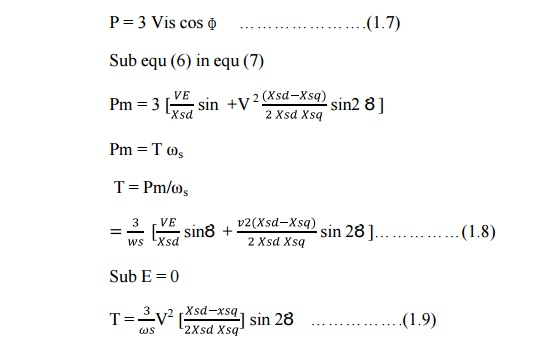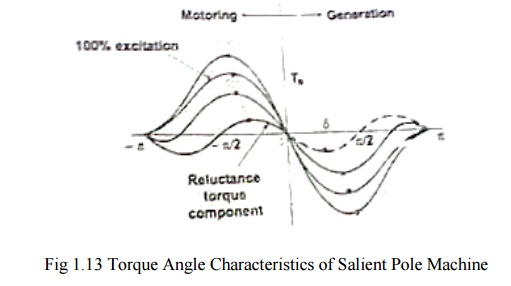Home | | Special Electrical Machines | Phaser Diagram of Synchronous Reluctance Motor

# Phaser Diagram of Synchronous Reluctance Motor

The synchronous reluctance machine is considered as a balanced three phase circuit, it is sufficient to draw the phasor diagram for only one phase.

PHASER DIAGRAM OF SYNCHRONOUS RELUCTANCE MOTOR

The synchronous reluctance machine is considered as a balanced three phase circuit, it is sufficient to draw the phasor diagram for only one phase. The basic voltage equation neglecting the effect of resistance isV = E – j IsdXsd – j Isq…………(1.1)

Where

V is the Supply Voltage

Is is the stator current

E is the excitation emf

ɸ is the phase angle

Xsd and Xsq are the synchronous reactance of direct and quadrature axis

Isd and Isq are the direct and quadrature axis current

I = Isd + Isq…………….(1.2)

Isd is in phase quadrtur with E and Isq is in phase with E.

V = E – j IsdXsd – j IsqXsqEquation (9) is the torque equation of synchronous reluctance motor.Plotting the equation (9) as shown in fig indicates that the stability limit is reached at Ȣ /4

And by increasing g load angle torque also increases.In synchronous reluctance motor, the excitation emf(E) is zero.Study Material, Lecturing Notes, Assignment, Reference, Wiki description explanation, brief detail
Special Electrical Machines : Synchronous Reluctance Motors : Phaser Diagram of Synchronous Reluctance Motor |